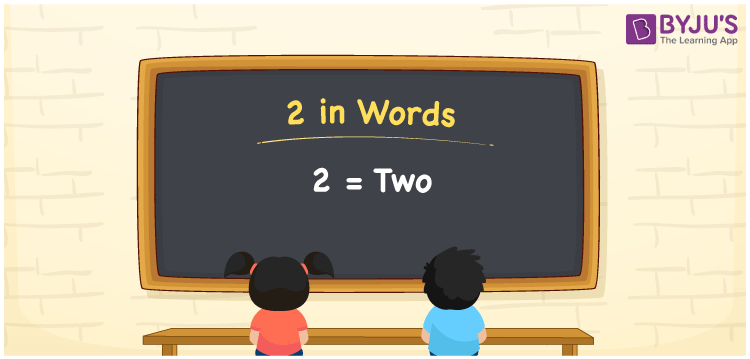# 2 in Words

2 in words is written as “Two”. Number 2 is used to express a value or a count of objects. It comes at the 2nd number in a set of natural numbers. The spelling or name of 2 in English is given by Two. For example, there are 2 pens kept in a pen-stand. Hence, it is necessary to learn the numbers in words, to read and write and express them.

 2 in words Two Two in Numbers 2

## 2 in English Words## How to Write 2 in Words?

This page discusses the concept of writing 2 in words. In the number 2, one digit is present. The table can be used to understand the place value chart for the one digit of the number 2.

 Ones 2

The number 3 using expanded form can be written as:

2 × One

= 2 × 1

= 2

= Two

Therefore, 2 in words is written as Two.

2 is a natural number that precedes 3 and succeeds 1.

2 in words – Two

Is 2 an odd number? – No

Is 2 an even number? – yes

Is 2 a perfect square number? – No

Is 2 a perfect cube number? – No

Is 2 a prime number? – Yes

Is 2 a composite number? – No

## Frequently Asked Questions on 2 in Words

Q1

### How can the number 2 be written in words?

2 can be written in words as “Two”.
Q2

### Is 2 an odd number?

No, 2 is an even number as it is divisible by 2.
Q3

### How can we write Two in numbers?

Two can be written in numbers as 2.